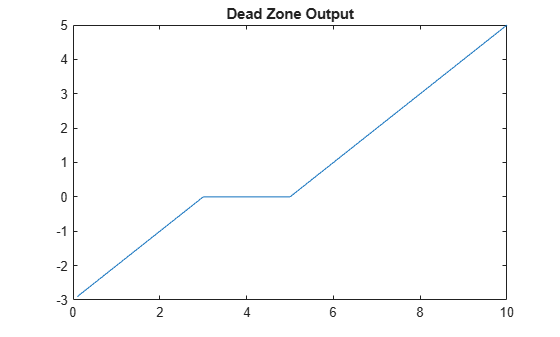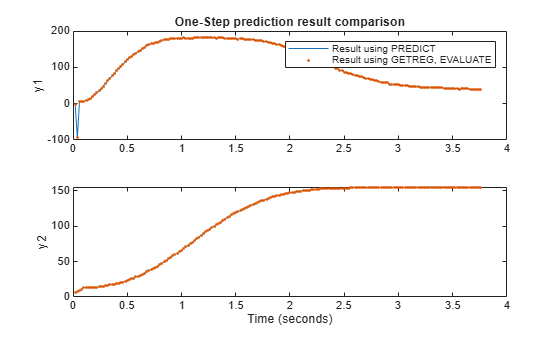# evaluate

Evaluate output values of `idnlarx` or `idnlhw` mapping object array for given set of input values

## Syntax

``Value = evaluate(MO,X)``
``Value = evaluate(MO,X,varnames)``

## Description

example

````Value = evaluate(MO,X)` computes the value of a mapping object array `MO` that contains objects such as `idWaveletNetwork` objects for the inputs in `X`. For a list of available mapping objects, see Available Mapping Functions for Nonlinear ARX Models or Available Nonlinearity Estimators for Hammerstein-Wiener Models. Use this syntax when you want to independently evaluate the output of a mapping object array that you are using to estimate an `idnlarx` or `idnlhw` model.```

example

````Value = evaluate(MO,X,varnames)` specifies the list of variable names `varnames` that orders the input data for the `MO.Inputs` property. Use this syntax when the columns of data in `X` are not in the order that the nonlinear model blocks require.```

## Examples

collapse all

Create a linear input signal `u` with slope `m`.

```m = 0.1; u = m*[1:100]';```

Create an `idDeadZone` object with a dead zone between 3 and 5.

```MO = idDeadZone; MO.ZeroInterval = [3 5]```
```MO = Dead Zone Inputs: u(t) Output: y(t) Inputs: {'u(t)'} Outputs: {'y(t)'} ZeroInterval: [3 5] Free: [1 1] ```

Evaluate the output of MO using `u` as the input.

```e = evaluate(MO,u); plot(u,e) title('Dead Zone Output')```The plot shows a zone of no response between 3 and 5.

Load the data, which contains input matrix `u` and output matrix `y`. `u` contains data for six inputs and `y` contains data for two outputs. Encapsulate `u` and `y` in an `iddata` object with a sample time of 0.02 sec.

```load motorizedcamera z = iddata(y,u,0.02,'Name','Motorized Camera','Timeunit','s');```

Estimate `idnlarx` Model and Predict Model Output

Specify the order `nn` for the `nlarx` estimation.

```na = [2 1;1 4]; nb = [1 4 2 2 1 3;3 3 3 1 2 4]; nc = [ones(2,3),zeros(2,3)]; nn = [na nb nc];```

Estimate an `idnlarx` model after first turning off the normalization option. Specify two different mapping objects for the output function.

```opt = nlarxOptions('Normalize',false); sys = nlarx(z, nn, [idWaveletNetwork(3); idSupportVectorMachine('linear')],opt)```
```sys = Nonlinear ARX model with 2 outputs and 6 inputs Inputs: u1, u2, u3, u4, u5, u6 Outputs: y1, y2 Regressors: Linear regressors in variables y1, y2, u1, u2, u3, u4, u5, u6 Output functions: Output 1: Wavelet network with 3 units Output 2: Support Vector Machine function using a Linear kernel Sample time: 0.02 seconds Status: Estimated using NLARX on time domain data "Motorized Camera". Fit to estimation data: [98.87;91.79]% (prediction focus) FPE: 23.94, MSE: 22.44 More information in model's "Report" property. ```

Predict the model output using one-step-ahead prediction and store the result in `yp`.

```yp = predict(sys,z,1,predictOptions('InitialCondition','zero')); yp = yp.OutputData;```

Use `evaluate` to Map Regressors to Mapping Object Output

Use `getreg` to compute and store the regressor data in `D`.

```D = getreg(sys,z); D = D{:,:};```

The two mapping objects, `y1` and `y2`, use different regressors. Because of this difference, `evaluate` requires the regressor names to order the regressors correctly for the object inputs. Use `getreg` to retrieve the regressor names and store them in `regnames`. View the first five regressor names.

```regnames = getreg(sys); regnames(1:5)```
```ans = 5x1 cell {'y1(t-1)'} {'y1(t-2)'} {'y2(t-1)'} {'y2(t-2)'} {'y2(t-3)'} ```

Evaluate the output of `sys.OutputFcn` using the regressor data and names.

`yev = evaluate(sys.OutputFcn,D,regnames);`

Compare Output Results

Plot the estimated and evaluated results for the two model outputs `y1` and `y2`.

```tiledlayout(2,1) nexttile t = z.SamplingInstants; plot(t,yp(:,1),t,yev(:,1),'.') ylabel('y1') title('One-Step prediction result comparison') legend('Result using PREDICT','Result using GETREG, EVALUATE') nexttile plot(t,yp(:,2),t,yev(:,2),'.') ylabel('y2') xlabel('Time (seconds)')```The `predict` and `evaluate` results are essentially the same.

## Input Arguments

collapse all

Mapping object array, specified as a single mapping object or an array of length ny, where ny is the number of both mapping objects and outputs. For an `idnlarx` model `sys`, `MO` represents the `sys.OutputFcn` property. For an `idnlhw` model `sys`, `MO` represents the `sys.InputNonlinearity` or `sys.OutputNonlinearity` property.

Input values at which to evaluate `MO`, specified as a single numeric value or an nv-by-nx matrix, where nv is the number of points at which to evaluate `MO` and nx is the number of inputs to `MO`. For nonlinear ARX models, the inputs are the input regressor signals. For Hammerstein-Wiener models, the inputs are either the true input signals (input nonlinearity) or the outputs of the linear block (output nonlinearity).

Variable names associated with each column of `X`, specified as a string array of length nx, where nx is the number of input columns. `varnames{i}` corresponds to `X(:,i)`. The software uses `varnames` to order the data columns in accordance with the property `MO.Inputs`. If you do not specify `varnames`, then the columns are ordered monotonically. Therefore, omit specifying `varnames` only when the columns of `X` are already in the same order as in `MO.Inputs`.

## Output Arguments

collapse all

Evaluated values, returned as a numeric value or an nv-by-ny array of numeric values, where nv is the number of points to evaluate and ny is the number of outputs.

## Version History

Introduced in R2007a